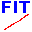#QtiSAS|QtiKWS

SANS: reduction, analysis, global instrumental fit

### Sidebar

#### JCNS :: Institutes

compile:sasview:sphere:core-multi-shell# Sasview Function:Core Multi Shell

Implementation of “Core Multi Shell” fitting Function of SASVIEW. SASVIEW reference is here: http://marketplace.sasview.org/models/19/.

(1)            $I(q)=\frac{\phi}{V(R_N)}F^2(q)\cdot10^{-4}$            $[cm^{-1}]$

(2)            $F(q) = \sum_{i=0}^N V(R_{i})(\rho_{i+1}-\rho_i) \cdot \frac {3 \cdot j_1(qR_i)}{qR_i}$

(3)            $V(R)=\frac{4 \cdot \pi}{3}R^3$

(4)            $N=3$

 $i=0$ $i=1$ $\rho_0=\rho_{core}$ $R_0=R_{core}$ $\rho_1=\rho_{shell-No.-1}$ $R_1=R_{core}+\Delta_1$ $\rho_2=\rho_{shell-No.-2}$ $R_2=R_{core}+\Delta_1+\Delta_2$ … $\rho_N=\rho_{shell-No.-N}$ $R_N=R_{core}+\sum_{j=1}^N\Delta_j$ $\rho_{N+1}=\rho_{solvent}$ —

c-code of SASVIEW “core_multi_shell.c” is saved as the header file sasview-core_multi_shell.h.

Full function (with volume fraction and normalised to the volume) is also implemented as the header-file sasview-multicoreshell-3-shells.h. This implementation could be used as a sub-function of a more complicated function.

Finally, standard fif-function is created: sphere-core-3x-shell.fif.

Compilation:

• download 4 header files below and save them to your qtitisas-folder: ./qtisas/FitFunctions/IncludedFunctions/ folder
• download sphere-core-3x-shell.fif file and save it to ./qtisas/FitFunctions/ folder
• select “sphere-core-3x-shell” function in Fit.Compile interface and compile it.
 h-files: FIF-file: sasview.h Copy h-files to ./qtisas/FitFunctions/IncludedFunctions/ folder sasview-sas_3j1x_x.h Spherical Bessel function: $\frac {3 \cdot j_1(x)}{x}$ sasview-core_multi_shell.h sasview-multicoreshell-3-shells.h sphere-core-3x-shell.fif Copy fif-file to ./qtisas/FitFunctions/ folder
compile/sasview/sphere/core-multi-shell.txt · Last modified: 2021/03/22 17:41 by Vitaliy Pipich

### Page Tools